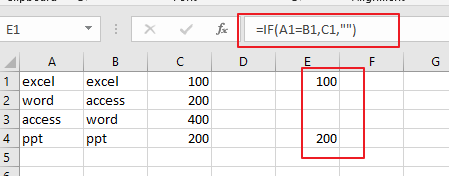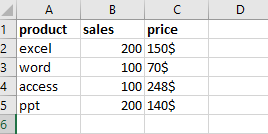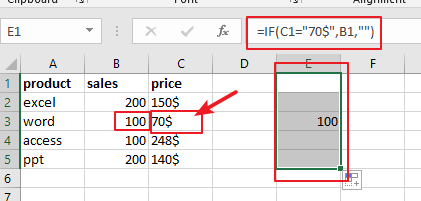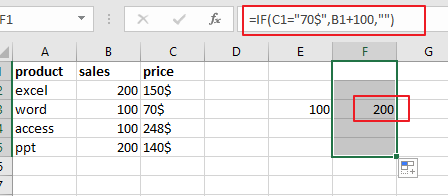# How to Get Another Cell Value If One Cell Equals in Excel

This post will guide you how to extract another cell value if one specified cell equals to a given value or an adjacent cell value using a formula in Excel 2013/2016. Normally, if you want to do some operation when a given cell equals a certain value, and you can create a new formula based on IF function to test that value, then do something if the test result is TRUE, otherwise, do something else if the test result is FALSE.

Assuming that you have a list of data in range A1:C5, and you want to extract cell values from column C when the corresponding cell value in Column A equals the adjacent cell values in Column B, if True, return the corresponding Cell value in Column C. How to achieve it.

You can use the following formula:

=IF(A1=B1,C1,””)

You need to enter the above formula into a blank cell and then drag it down to other cells to apply this formula.Note: Cell A1 and Cell B1 are the two cells that you wish to compare, and Cell C1 is the cell value that you want to extract.

There is another example based on the following data table, you just simply want to get cell Value in Column B when the Cell value in Column C equals to `“70\$`”.You can use the below formula:

=IF(C1=”70\$”,B1,””)

The logic test for the above formula is:

C1=”70\$”

This will return True Value if Cell C1 value equals a certain value “`70\$`”, otherwise, returns FALSE.We only need to take action when the result of the logic test is TRUE. And here it will return the corresponding cell value in Column B when the cell value in Column C equals “70\$”. And if the cell value is not “70\$”, it will return an empty string.

For the above formula, if you did not specify that empty string when the logic test is FALSE, and the formula would display FALSE whenever the Cell value is not “70\$”.

Actually, you can also do something more complicated operation as well. For example, you wish to increase the sales when the price is equal to “70\$” by 100. In this case, you can could use the below formula based on IF function:

=IF(C1=”70\$”,B1+100,””)

From the above screenshot, and you would see that the original value in Column B has been increased by 100 when the cell value in Column C is “70\$”.### Related Functions

• Excel IF function
The Excel IF function perform a logical test to return one value if the condition is TRUE and return another value if the condition is FALSE. The IF function is a build-in function in Microsoft Excel and it is categorized as a Logical Function.The syntax of the IF function is as below:= IF (condition, [true_value], [false_value])….
Related Posts

Calculate Days Open in Excel

If you want to know how to Calculate days in Excel, there are some formulas that you can use to do so. For example, you can use the DAYS function in Excel to find the number of days between two ...

Add Row Numbers And Skip Blanks in Excel

Do you ever have to input a list of numbers into a spreadsheet, and some of the cells are blank? It can be difficult to keep track of what number is in which cell when you have to scroll up ...

Assigning Points based on Late Time

It is shown in this lesson how to utilize the IF function in Excel to allocate points based on the amount of time that has passed. If you intend to pursue along this guide, you may do so by downloading ...

Check Dates in chronological order

Assume you have a date list that has different date formats, as seen in the accompanying picture. In this instance, Excel's Sort function will fail to sort them appropriately. However, you may convert all various date formats to a particular ...

Check If a Cell is Blank or Empty

The article demonstrates how to check if a range of cells is blank or empty by ISBLANK and other functions to recognize empty cells in Excel and take appropriate action based on their status. There are several circumstances in which ...

Abbreviate Names Or Words in Excel

As an MS Excel user, you might have come across a task where you need to abbreviate different names or words, and there are also possibilities that you might have done this task manually by assuming that there isn't any ...

Extract or Filter on The Top N Values with Criteria

Suppose that you are in a situation where you need to filter out the top n values from the list having few values with specific criteria, or you can say that with a particular condition, and I am also pretty ...

Extract matching values From Two Lists

Suppose that you are working with two lists containing few values, and you want to extract the matching values from those two lists into another separate list. You might prefer to manually extract the matching values from the two lists, ...

Extract Multiple Match Values into Separate Columns

If you have a few values/items in the excel sheet and you are thinking that with the aid of the “VlOOKUP” function you can look for a specific value, extract it and then put the matching item into the separate ...

VLOOKUP Formula | Faster Trick with 2 VLOOKUPS

This post will guide you how to use 2 VLOOKUPS function to looking up data entries from a given range of cells in Microsoft Excel. VLOOKUP with 2 lookups can be faster than a single VLOOKUP in certain scenarios. The ...

Sidebar